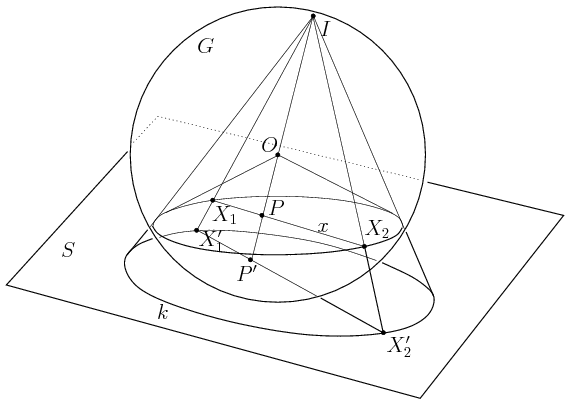Mathematical and Physical Journal
for High Schools
Issued by the MATFUND Foundation
 Already signed up? New to KöMaL?

#Problem A. 560. (April 2012)

A. 560. Given a right circular cone with vertex O, and a fixed point P in the base plane of the cone. Draw a line x through P which intersects the base circle of the cone at two points, X1 and X2. Show thatdoes not depend on the choice of the line x.

(5 pont)

Deadline expired on May 10, 2012.

Solution. Let G be the sphere with center O that contains the base circle of the cone. Let P' be the intersection of G with the ray OP, and let I be the point of G, diametrically opposite with P'.

Apply inversion to the sphere with center I and radius IP'. The image of G is the tangent plane S at point P'. The image of the base circle is some circle k in the plane S.Let X1' and X2' be the images of X1 and X2, respectively. The points P',X1',X2' are collinear, moreover X1',X2' lie on k. ThenThe numerator, being the power of P' with respect to k, does not depend on the points X1',X2'.

### Statistics:

 8 students sent a solution. 5 points: Ágoston Tamás, Janzer Olivér, Mester Márton, Omer Cerrahoglu, Strenner Péter, Szabó 789 Barnabás, Szabó 928 Attila. 4 points: Gyarmati Máté.

Problems in Mathematics of KöMaL, April 2012